# Algebra II : Number Theory

## Example Questions

← Previous 1 3 4 5 6 7 8 9 10

### Example Question #1 : Real Numbers

Using the properties of negative numbers, determine which answer is equivalent to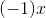Possible Answers:

All of the other answers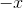Correct answer:Explanation:

Recall the following property of negative numbers: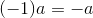It follows that: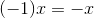### Example Question #2 : Real Numbers

Using the properties of negative numbers, determine which answer is equivalent to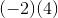Possible Answers: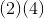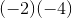All of the other answers

Correct answer:Explanation:

Recall the following property of negative numbers: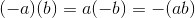It follows that: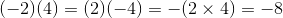### Example Question #3 : Real Numbers

Using the properties of negative numbers, determine which answer is equivalent to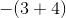Possible Answers: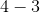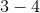All of the other answersCorrect answer:Explanation:

Recall the following property of negative numbers: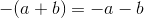It follows that: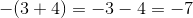### Example Question #4 : Real Numbers

Using the properties of negative numbers, determine which answer is equivalent to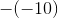Possible Answers: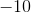All of the other answers

Correct answer:Explanation:

Recall the following property of negative numbers: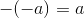It follows that: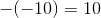### Example Question #5 : Real Numbers

Identify the property of real numbers represented by the following: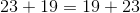Possible Answers:

Commutative Property of Addition

Associative Property of Multiplication

Associative Property of Addition

Commutative Property of Multiplication

Distributive Property

Correct answer:

Commutative Property of Addition

Explanation:

Recall that the Commutative Propery of Addition states: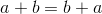It follows that:To help you tell the difference between commutative, associative, and distributive just remember what the words themselves mean.

When you commute you go from one place to another. With commutative properties, numbers are moving from one position to another.

When you associate with someone you are connected or grouped with them. The associative properties involve moving parentheses so that different numbers are grouped together, but they are not changing positions.

When you distribute things you are handing them out. With the distributive property, the number outside the parentheses is being handed out to the numbers inside the parentheses.

### Example Question #6 : Real Numbers

Identify the property of real numbers represented by the following: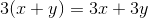Possible Answers:

Commutative Property of Multiplication

Associative Property of Addition

Associative Property of Multiplication

Distributive Property

Commutative Property of Addition

Correct answer:

Distributive Property

Explanation:

Recall that the Distributive Property states: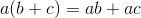It follows that:To help you tell the difference between commutative, associative, and distributive just remember what the words themselves mean.

When you commute you go from one place to another. With commutative properties, numbers are moving from one position to another.

When you associate with someone you are connected or grouped with them. The associative properties involve moving parentheses so that different numbers are grouped together, but they are not changing positions.

When you distribute things you are handing them out. With the distributive property, the number outside the parentheses is being handed out to the numbers inside the parentheses.

### Example Question #1 : Real Numbers

Identify the property of real numbers represented by the following: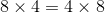Possible Answers:

Commutative Property of Multiplication

Associative Property of Addition

Commutative Property of Addition

Distributive Property

Associative Property of Multiplication

Correct answer:

Commutative Property of Multiplication

Explanation:

Recall that the Commutative Property of Multiplication states: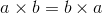It follows that :To help you tell the difference between commutative, associative, and distributive just remember what the words themselves mean.

When you commute you go from one place to another. With commutative properties, numbers are moving from one position to another.

When you associate with someone you are connected or grouped with them. The associative properties involve moving parentheses so that different numbers are grouped together, but they are not changing positions.

When you distribute things you are handing them out. With the distributive property, the number outside the parentheses is being handed out to the numbers inside the parentheses.

### Example Question #1 : Real Numbers

Identify the property of real numbers represented by the following: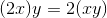Possible Answers:

Commutative Property of Addition

Distributive Property

Associative Property of Multiplication

Associative Property of Addition

Commutative Property of Multiplication

Correct answer:

Associative Property of Multiplication

Explanation:

Recall that the Associative Property of Multiplication states: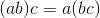It follows that:To help you tell the difference between commutative, associative, and distributive just remember what the words themselves mean.

When you commute you go from one place to another. With commutative properties, numbers are moving from one position to another.

When you associate with someone you are connected or grouped with them. The associative properties involve moving parentheses so that different numbers are grouped together, but they are not changing positions.

When you distribute things you are handing them out. With the distributive property, the number outside the parentheses is being handed out to the numbers inside the parentheses.

### Example Question #9 : Real Numbers

Identify the property of real numbers represented by the following: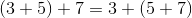Possible Answers:

Distributive Property

Commutative Property of Multiplication

Associative Property of Addition

Associative Property of Multiplication

Commutative Property of Addition

Correct answer:

Associative Property of Addition

Explanation:

Recall that the Associative Property of Addition states: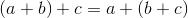It follows that:To help you tell the difference between commutative, associative, and distributive just remember what the words themselves mean.

When you commute you go from one place to another. With commutative properties, numbers are moving from one position to another.

When you associate with someone you are connected or grouped with them. The associative properties involve moving parentheses so that different numbers are grouped together, but they are not changing positions.

When you distribute things you are handing them out. With the distributive property, the number outside the parentheses is being handed out to the numbers inside the parentheses.

### Example Question #1 : Real Numbers

Identify the property of real numbers represented by the following: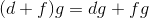Possible Answers:

Associative Property of Addition

Commutative Property of Multiplication

Associative Property of Multiplication

Commutative Property of Addition

Distributive Property

Correct answer:

Distributive Property

Explanation:

Recall that the Distributive Property states:The number outside the parentheses can be on the left or right side of the parentheses, so the following is also true: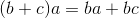It follows that:To help you tell the difference between commutative, associative, and distributive just remember what the words themselves mean.

When you commute you go from one place to another. With commutative properties, numbers are moving from one position to another.

When you associate with someone you are connected or grouped with them. The associative properties involve moving parentheses so that different numbers are grouped together, but they are not changing positions.

When you distribute things you are handing them out. With the distributive property, the number outside the parentheses is being handed out to the numbers inside the parentheses.

← Previous 1 3 4 5 6 7 8 9 10

### All Algebra II Resources